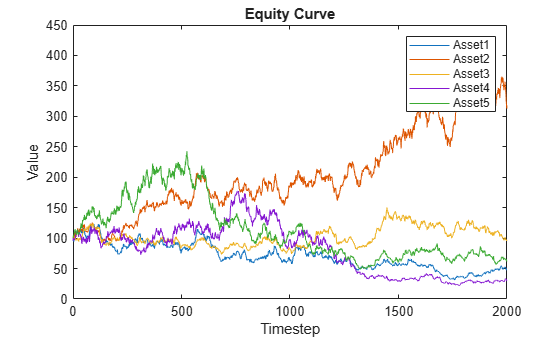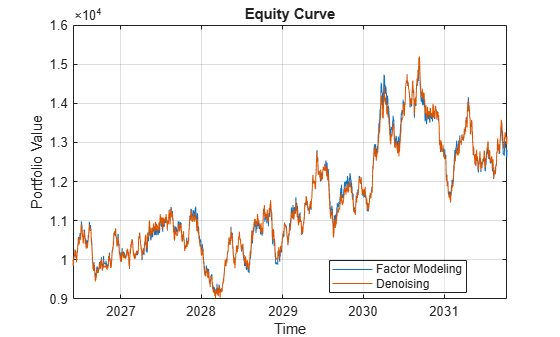# Compare Performance of Covariance Denoising with Factor Modeling Using Backtesting

This example uses backtesting to compare the performance of two investment strategies that use factor information to compute the portfolio weights. The first investment strategy uses `covarianceDenoising` to estimate both the covariance matrix and the number of factors to use in the second investment strategy. The second investment strategy uses a principal component analysis (PCA) factor model to estimate the covariance matrix with the number of factors obtained with `covarianceDenoising`. The PCA factor model follows the process in Portfolio Optimization Using Factor Models.

Load a simulated data set that includes asset returns for a total $\mathit{n}=100$ assets and 2000 daily observations.

```load('asset_return_100_simulated.mat'); [numObservations,numAssets] = size(stockReturns)```
```numObservations = 2000 ```
```numAssets = 100 ```

Create a timetable of asset prices from the asset returns.

```% Convert the returns to prices pricesT = ret2tick(stockReturns,'StartPrice',100); % Create timetable rowTimes = datetime("today"):datetime("today")+numObservations; pricesTT = table2timetable(pricesT,'RowTimes',rowTimes);```

Visualize the equity curve for each stock. For this example, plot the first five stocks.

```figure; plot(0:2000,pricesTT{:,1:5}) xlabel('Timestep'); ylabel('Value'); title('Equity Curve'); legend(pricesTT.Properties.VariableNames(1:5));```### Optimize Asset Allocation Using Covariance Denoising

Covariance denoising is a technique that you can use to reduce the noise and enhance the signal in a covariance matrix. First, the eigenvalues that are associated with noise are separated from the eigenvalues associated with signal. Then, the eigenvalues associated with noise are shrunk towards a target value. This technique helps improve the stability of the covariance matrix over time as well as its condition number.

The function `covarianceDenoising` computes the denoised estimate of the covariance matrix and returns as a second output the number of eigenvalues identified with signal. You use this number in the Optimize the Asset Allocation Using Factor Modeling section to determine the number of factors that the factor model allocation uses.

This example uses the first 42 days (approximately 2 months) of the data set to select the initial portfolio allocations.

```% Warm-up period warmupPeriod = 42; % No current weights (100% cash position) w0 = zeros(1,numAssets); % Warm-up partition of prices timetable warmupTT = pricesTT(1:warmupPeriod,:);```

Compute the maximum return portfolio subject to a target risk of `0.008` using the denoised covariance estimate.

```% Compute weights with denoised strategy wDenoised_initial = denoising(w0,warmupTT);```

Check for asset allocations that are over 5% to identify assets with large investment weights.

```percentage = 0.05; AssetName = pricesTT.Properties.VariableNames(... wDenoised_initial>=percentage)'; Weight = wDenoised_initial(wDenoised_initial>=percentage); T1 = table(AssetName,Weight)```
```T1=5×2 table AssetName Weight ___________ ________ {'Asset6' } 0.066014 {'Asset47'} 0.10991 {'Asset50'} 0.24654 {'Asset75'} 0.11752 {'Asset94'} 0.31708 ```

### Optimize Asset Allocation Using Factor Modeling

For factor modeling, you can use statistical factors extracted from the asset return series. In this example, PCA is used to extract these factors . You can then use this factor model to solve the portfolio optimization problem.

With a factor model, $\mathit{n}$ asset returns can be expressed as a linear combination of $\mathit{k}$ factor returns, ${\mathit{r}}_{\mathit{a}}={\mu }_{\mathit{a}\text{\hspace{0.17em}}}+\mathit{F}\text{\hspace{0.17em}}{\mathit{r}}_{\mathit{f}}+{\epsilon }_{\mathit{a}}\text{\hspace{0.17em}}$, where $\mathit{k}\ll \mathit{p}$. In the mean-variance framework, portfolio risk is

$\mathrm{Var}\left({\mathit{R}}_{\mathit{p}}\right)=\mathrm{Var}\left({{\mathit{r}}_{\mathit{a}}}^{\mathit{T}}{\mathit{w}}_{\mathit{a}}\right)=\mathrm{Var}\left({\left({\mu }_{\mathit{a}\text{\hspace{0.17em}}}+\mathit{F}\text{\hspace{0.17em}}{\mathit{r}}_{\mathit{f}}+{\epsilon }_{\mathit{a}}\right)}^{\mathit{T}}{\mathit{w}}_{\mathit{a}}\right)={\mathit{w}}_{\mathit{a}}^{\mathit{T}}\left(\mathit{F}{\Sigma }_{\mathit{f}}{\mathit{F}}^{\mathit{T}}+\mathit{D}\right){\text{\hspace{0.17em}}\mathit{w}}_{\mathit{a}}$,

where:

• ${\mathit{R}}_{\mathit{p}}$ is the portfolio return (a scalar).

• ${\mathit{r}}_{\mathit{a}}$ is the asset returns.

• ${\mu }_{\mathit{a}\text{\hspace{0.17em}}}$ is the mean of asset returns.

• $\mathit{F}$ is the factor loading, with dimension $\mathit{n}×\mathit{k}$.

• ${\mathit{r}}_{\mathit{f}}$ is the factor return.

• ${\epsilon }_{\mathit{a}}$ is the idiosyncratic return related to each asset.

• ${\mathit{w}}_{\mathit{a}}$ is the asset weight.

• ${\Sigma }_{\mathit{f}}$ is the covariance of factor returns.

• $\mathit{D}$ is the variance of idiosyncratic returns.

The parameters ${\mathit{r}}_{\mathit{a}}$, ${\mathit{w}}_{\mathit{a}}$, ${\mu }_{\mathit{a}}$ and ${\epsilon }_{\mathit{a}}\text{\hspace{0.17em}}$are $\mathit{n}×\mathrm{1}$ column vectors, ${\mathit{r}}_{\mathit{f}}$and ${\mathit{w}}_{\mathit{f}}$ are $\mathit{k}×1$ column vectors, and ${\Sigma }_{\mathit{k}}$ and $\mathit{D}$ are a $\mathit{k}×\mathit{k}$ and a $\mathit{n}×\mathit{n}$ matrices, respectively.

Therefore, the mean-variance optimization problem is formulated as

`$\begin{array}{l}\underset{{\mathit{w}}_{\mathit{a}}}{\mathrm{max}}\text{\hspace{0.17em}\hspace{0.17em}\hspace{0.17em}}{\mu }_{\mathit{a}}^{\mathit{T}}{\mathit{w}}_{\mathit{a}}\\ \mathit{s}.\mathit{t}.\text{\hspace{0.17em}\hspace{0.17em}}{\mathit{w}}_{\mathit{a}}^{\mathit{T}}\left(\mathit{F}{\Sigma }_{\mathit{f}}{\mathit{F}}^{\mathit{T}}+\mathit{D}\right){\mathit{w}}_{\mathit{a}}\le \tau ,\\ \text{\hspace{0.17em}\hspace{0.17em}\hspace{0.17em}\hspace{0.17em}\hspace{0.17em}\hspace{0.17em}\hspace{0.17em}\hspace{0.17em}\hspace{0.17em}\hspace{0.17em}}\sum _{\mathit{a}\in \mathit{A}}{\mathit{w}}_{\mathit{a}}=1,\\ \text{\hspace{0.17em}\hspace{0.17em}\hspace{0.17em}\hspace{0.17em}\hspace{0.17em}\hspace{0.17em}\hspace{0.17em}\hspace{0.17em}\hspace{0.17em}\hspace{0.17em}}0\le {\mathit{w}}_{\mathit{a}}\le 1.\end{array}$`

In the dimensional space formed by $\mathit{n}$ asset returns, PCA finds the $\mathit{k}$ directions that capture the most important variations in the returns. Usually, $\mathit{k}$ is less than $\mathit{n}$. Therefore, by using PCA, you can decompose the $\mathit{n}$ asset returns into $\mathit{k}$ directions that are interpreted as factor loadings. The scores from the decomposition are interpreted as the factor returns. For more information, see `pca` (Statistics and Machine Learning Toolbox™). In this example, the factor model uses $\mathit{k}=$`nFactors`, where `covarianceDenoising` determines the `numFactors`.

Compute the maximum return portfolio subject to a target risk of 0.008 using the factor model covariance estimate. For details on how to obtain the weights allocation using factor modeling, see Portfolio Optimization Using Factor Models.

```% Compute weights with denoised strategy userData.numFactors = []; [wFactorModel_initial,userData] = factorModeling(w0,warmupTT, ... userData);```

Check for asset allocations that are over 5% to show assets with large investment weights.

```percentage = 0.05; AssetName = pricesTT.Properties.VariableNames( ... wFactorModel_initial>=percentage)'; Weight = wFactorModel_initial(wFactorModel_initial>=percentage); T2 = table(AssetName,Weight)```
```T2=6×2 table AssetName Weight ___________ ________ {'Asset6' } 0.075366 {'Asset35'} 0.069395 {'Asset47'} 0.10676 {'Asset50'} 0.21628 {'Asset75'} 0.12423 {'Asset94'} 0.3068 ```

The assets with large investment weights are almost the same for both investment strategies. `Asset35` is the only asset that appears in one table, namely in the factor model strategy, and not the other. Even the weights of the assets are similar.

### Backtesting

Use `backtestStrategy` to create strategy objects for the two investment strategies. Compare the denoising strategy (`strat1`) against the factor model strategy (`strat2`) using backtesting.

```% Rebalance approximately every month rebalFreq = 21; % Set the rolling lookback window to be at least 2 months and at % most 6 months lookback = [42 126]; % Use a fixed transaction cost (buy and sell costs are both 0.5% % of amount traded) transactionsFixed = 0.005; % Strategies strat1 = backtestStrategy('Factor Modeling', @factorModeling, ... UserData=userData, ... RebalanceFrequency=rebalFreq, ... LookbackWindow=lookback, ... TransactionCosts=transactionsFixed, ... InitialWeights=wFactorModel_initial); strat2 = backtestStrategy('Denoising', @denoising, ... RebalanceFrequency=rebalFreq, ... LookbackWindow=lookback, ... TransactionCosts=transactionsFixed, ... InitialWeights=wDenoised_initial); % Aggregate the strategy objects into an array strategies = [strat1, strat2];```

Create a `backtestEngine` object for the strategies, run the backtest using `runBacktest`, and generate a report using `summary`.

```% Create the backtesting engine object backtester = backtestEngine(strategies); % Run backtest backtester = runBacktest(backtester,pricesTT,'Start',warmupPeriod); % Generate summary table of strategies performance summary(backtester)```
```ans=9×2 table Factor_Modeling Denoising _______________ __________ TotalReturn 0.28079 0.31501 SharpeRatio 0.018583 0.020462 Volatility 0.0089592 0.0086696 AverageTurnover 0.014646 0.013843 MaxTurnover 0.56184 0.58224 AverageReturn 0.00016644 0.00017736 MaxDrawdown 0.23384 0.24536 AverageBuyCost 0.84446 0.79536 AverageSellCost 0.84446 0.79536 ```

Use `equityCurve` to plot the equity curve to compare the performance of both strategies.

`equityCurve(backtester)`The performance of both strategies is similar, although not identical. This similarity is because the factor model strategy uses the number of factors identified by `covarianceDenoising` to select the number of principal components. Factor model strategies are usually not implemented this way, but rather the number of factors is a fixed parameter that is chosen a priori.

In this example, the number of factors that is most frequently identified is `1`.

```% Count the number of different factors categoricalNumFactors = ... categorical(backtester.Strategies(1).UserData.numFactors); [N,uniqueFactors] = histcounts(categoricalNumFactors); factorFrequency = table(uniqueFactors',N', ... 'VariableNames',{'NumFactors','Frequency'})```
```factorFrequency=3×2 table NumFactors Frequency __________ _________ {'1' } 81 {'2' } 12 {'17'} 1 ```

Therefore, you can run the factor modeling strategy using `1` as the number of factors instead of using `covarianceDenoising` to identify the number of factors at each rebalancing period.

### Reference

1. Meucci, Attilio. “Modeling the Market.” In Risk and Asset Allocation, by Attilio Meucci, 101–66. Berlin, Heidelberg: Springer Berlin Heidelberg, 2009.

#### Local Functions

```function [new_weights,userData] =... factorModeling(~,pricesTT,userData) % Compute minimum variance portfolio using traditional covariance estimate. % Compute returns from prices timetable. assetReturns = tick2ret(pricesTT); % Compute the number of factors identified using covariance denoising. [~,numFactors] = covarianceDenoising(assetReturns.Variables); userData.numFactors = [userData.numFactors; numFactors]; % Compute the covariance using the factors model % SigmaFactorModel = F*Sigma_f*F' + D % r_a = mu_a + F*r_f + epsilon_a [factorLoading,factorRetn,~,~,~,factorMean] = ... pca(assetReturns.Variables,'NumComponents',numFactors); covFactor = cov(factorRetn); retnHat = factorRetn*factorLoading' + factorMean; unexplainedRetn = assetReturns.Variables - retnHat; unexplainedCovar = diag(cov(unexplainedRetn)); D = diag(unexplainedCovar); % Define the mean and covariance of the returns. mu = mean(assetReturns.Variables); Sigma = factorLoading*covFactor*factorLoading' + D; % Create the portfolio problem. p = Portfolio(AssetMean=mu,AssetCovar=Sigma); % Specify long-only, fully-invested contraints p = setDefaultConstraints(p); % Compute the maximum return portfolio subject to the target risk. targetRisk = 0.008; new_weights = estimateFrontierByRisk(p,targetRisk); end function new_weights = denoising(~, pricesTT) % Compute minimum variance portfolio using covariance denoising. % Compute the returns from the prices timetable. assetReturns = tick2ret(pricesTT); mu = mean(assetReturns.Variables); Sigma = covarianceDenoising(assetReturns.Variables); % Create the portfolio problem. p = Portfolio(AssetMean=mu,AssetCovar=Sigma); % Long-only fully invested contraints p = setDefaultConstraints(p); % Compute maximum return portfolio subject to the target risk. targetRisk = 0.008; new_weights = estimateFrontierByRisk(p,targetRisk); end```